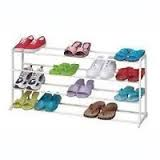## How many? Counting Stuff in Korean

Counting stuff primarily happens in Pure Korean (as opposed to Sino-Korean).

However, most other uses of numbers are Sino-Korean. This presents an interesting challenge when dealing with the following three questions:

1. How much?
2. How many?
3. How long?
• “How much?” is answered in Sino-Korean numbers.
얼마예요?” = “How much is it?” and a typical answer is “오천 원이예요.”
• “How many?” is answered in Pure Korean numbers AND requires a Counter.
있어요?” = “How many are there?” and a typical answer is “다섯 있어요.” (Here, is the counter. is the question word.)
• “How long?” is answered in Sino-Korean numbers.
얼마나 걸려요?” = “How long will it take?” and a typical answer is “분만 걸려요.” (This question uses the same 얼마 for “how much [time]” as the first question.)

To make matters even more confusing, 개 is not the only Counter in Korean. Rather, nearly anything that can be counted has its own Counter. For example, you can count:

1. People (분/명)
2. Animals (마리)
3. Books (권)
4. Bottles (병)
5. Papers (장)
6. Glasses (잔)
7. Cars/Appliances/Large Electronics (대)
8. Age (살)
9. Flowers (송이)
10. Shoes (켤레)

Additionally, the Pure Korean counting numbers are occasionally modified for smoother counting:

1. 하나 (1) becomes 한 개
2. 둘 (2) becomes 두 개
3. 셋 (3) becomes 세 개
4. 넷 (4) becomes 네 개
5. 스물 (20) becomes 스무 개

Hints:

1. All the other Pure Korean numbers (like 다섯, 여섯, etc) don’t change when counting.
2. Even if you can’t remember the specific Counter, you can use 개 as a default. It isn’t right, but you’ll be understood, especially while you’re learning Korean.
3. 몇 is the question word preceding all Counter questions. (“How many people?” = 몇 명/분? ; “How many things?” = 몇 개?)
4. Time in hours is counted (한 시; 한 시간), but time in minutes and seconds is spoken with Sino-Korean (이십오 분; 삼십 초).

## Table몇 개? 몇 분? 몇 명? 몇 마리? 몇 병? 몇 잔? 세 개 두 분 여섯 명 세 마리 세 병 네 잔몇 권? 몇 장? 몇 살? 몇 송이? 몇 켤레? 몇 대? 일곱 권 여덟 장 한 살 세 송이 열두 켤레 네 대

One more thing to note: positions are counted with 번째 or 째:

• 1st = 첫쩨/처 번째
• 2nd = 둘째/두 번째
• 3rd = 셋째/세 번째
• 4th = 넷째/네 번째
• 5th = 다섯 번째
• 6th = 여섯 번째
• 7th = 일곱 번째
• 8th = 여덟 번째
• 9th = 아홉 번째
• 10th = 열 번째
• 11th = 열한째
• 12th = 열두 번째
• etc…